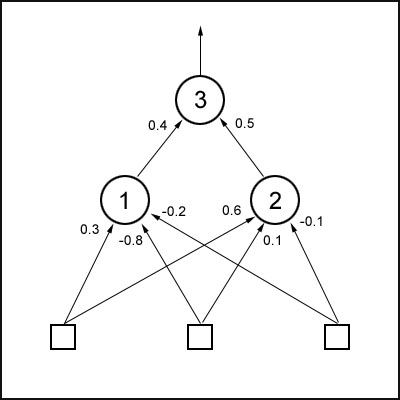The CGenAlg Class

This is the genetic algorithm class. If you followed my last tutorial you should have a good enough understanding of how they work. There is a difference with the CGenAlg class though because this time we are going to use vectors of real numbers instead of binary strings.

The neural network is encoded by reading all the weights from left to right and from the first hidden layer upwards and storing them in a vector. So if a network looked like this:The vector would be:   0.3, -0.8, -0.2, 0.6, 0.1, -0.1, 0.4, 0.5

(note, this is not taking into account the bias, just the weights as shown)

We can now use crossover and mutation as normal with one difference: the mutation rate for genetic algorithms using real numbers is much higher… a value of between 0.05 and 0.2 is recommended.

Before I show you the definition of the CGenAlg class let me quickly show you the genome structure:

 struct SGenome {   vector   vecWeights;     double           dFitness;     SGenome():dFitness(0){}     SGenome( vector w, double f): vecWeights(w), dFitness(f){}     //overload '<' used for sorting   friend bool operator<(const SGenome& lhs, const SGenome& rhs)   {     return (lhs.dFitness < rhs.dFitness);   } };

And now the CGenAlg class:

 class CGenAlg { private:   //this holds the entire population of chromosomes   vector m_vecPop;     //size of population   int m_iPopSize;     //amount of weights per chromo   int m_iChromoLength;     //total fitness of population   double m_dTotalFitness;     //best fitness this population   double m_dBestFitness;     //average fitness   double m_dAverageFitness;     //worst   double m_dWorstFitness;     //keeps track of the best genome   int m_iFittestGenome;     //probability that a chromosomes bits will mutate.   //Try figures around 0.05 to 0.3 ish   double m_dMutationRate;     //probability of chromosomes crossing over bits   //0.7 is pretty good   double m_dCrossoverRate;     //generation counter   int m_cGeneration;     void Crossover(const vector &mum,                  const vector &dad,                  vector       &baby1,                  vector       &baby2);     void Mutate(vector &chromo);     SGenome GetChromoRoulette();     void GrabNBest(int             NBest,                  const int       NumCopies,                  vector &vecPop);     void CalculateBestWorstAvTot();     void Reset();   public:   CGenAlg(int    popsize,           double MutRat,           double CrossRat,           int    numweights);     //this runs the GA for one generation.   vector Epoch(vector &old_pop);     //-------------------accessor methods   vector GetChromos()const{return m_vecPop;}   double AverageFitness()const{return m_dTotalFitness / m_iPopSize;}   double BestFitness()const{return m_dBestFitness;} };

When a CGenAlg object is created, the number of weights in each minesweeper's neural net is passed to it, along with the total population size. The constructor initializes the entire population with random weights and then each chromosome is allocated to its respective minesweepers 'brain' using the method CNeuralNet::PutWeights.

The minesweepers are then ready for action!

1  2  3  4  5  6  8  Next  Home